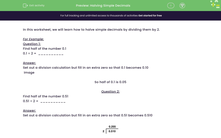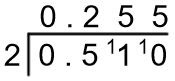# Halving Simple Decimals

In this worksheet, students practise halving simple decimals by adding extra zeros if necessary in the division calculation.Key stage:  KS 2

Curriculum topic:   Number: Fractions, Decimals and Percentages

Curriculum subtopic:   Divide with Two Decimal Places

Difficulty level:#### Worksheet Overview

In this worksheet, we will learn how to halve simple decimals by dividing them by 2.

For Example:

Question 1:

Find half of the number 0.1

0.1 ÷ 2 =  __________

Set out a division calculation but fill in an extra zero so that 0.1 becomes 0.10So half of 0.1 is 0.05

Question 2:

Find half of the number 0.51

0.51 ÷ 2 =  __________

Set out a division calculation but fill in an extra zero so that 0.51 becomes 0.510So half of 0.51 is 0.255

### What is EdPlace?

We're your National Curriculum aligned online education content provider helping each child succeed in English, maths and science from year 1 to GCSE. With an EdPlace account you’ll be able to track and measure progress, helping each child achieve their best. We build confidence and attainment by personalising each child’s learning at a level that suits them.

Get started